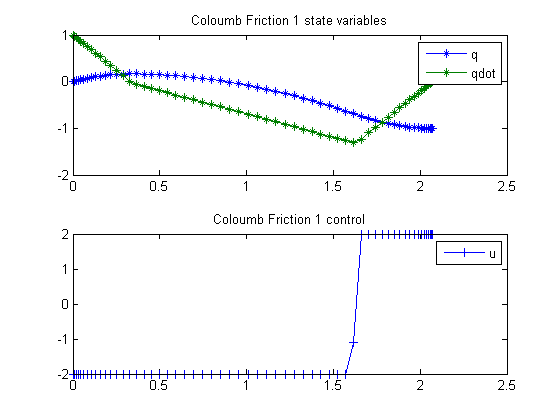25  Coloumb Friction 1

Minimum-Time Control of Systems With Coloumb Friction: Near Global Optima Via Mixed Integer Linear Programming, Brian J. Driessen, Structural Dynamics Department, Sandia National Labs.

4. Numerical Examples

25.1  Problem Formulation

Find u over t in [0; t_F ] to minimize

 J = tf

subject to:

 d2q dt2
= u − sign(
 dq dt
 −2 <= u <= 2
 q0 = 0
 dq dt
0 = 1
 q2 = −1
 dq dt
2 = 0

Reference: 

25.2  Problem setup

toms t
toms t_f

p = tomPhase('p', t, 0, t_f, 60);
setPhase(p);

tomStates q qdot
tomControls u

% Initial guess
x0 = {t_f == 1, icollocate(q == -t)};

% Box constraints
cbox = {-2 <= collocate(u) <= 2
0.001 <= t_f};

% Boundary constraints
cbnd = {initial({q == 0; qdot == 1}), final({q == -1, qdot == 0})};

% ODEs and path constraints
ceq = collocate({
dot(q)    == qdot
dot(qdot) == u-sign(qdot)});

objective = t_f;

25.3  Solve the problem

options = struct;
options.name = 'Coloumb Friction 1';
constr = {cbox, cbnd, ceq};
solution = ezsolve(objective, constr, x0, options);

t    = subs(collocate(p,t),solution);
q    = subs(collocate(p,q),solution);
qdot = subs(collocate(p,qdot),solution);
u    = subs(collocate(p,u),solution);
Problem type appears to be: lpcon
Starting numeric solver
===== * * * =================================================================== * * *
TOMLAB - Tomlab Optimization Inc. Development license  999001. Valid to 2011-02-05
=====================================================================================
Problem: ---  1: Coloumb Friction 1             f_k       2.070229757012032500
sum(|constr|)      0.000000001043060926
f(x_k) + sum(|constr|)      2.070229758055093200
f(x_0)      1.000000000000000000

Solver: snopt.  EXIT=0.  INFORM=1.
SNOPT 7.2-5 NLP code
Optimality conditions satisfied

FuncEv    1 ConstrEv  130 ConJacEv  130 Iter   23 MinorIter  676
CPU time: 0.609375 sec. Elapsed time: 0.641000 sec.

25.4  Plot result

subplot(2,1,1)
plot(t,q,'*-',t,qdot,'*-');
legend('q','qdot');
title('Coloumb Friction 1 state variables');

subplot(2,1,2)
plot(t,u,'+-');
legend('u');
title('Coloumb Friction 1 control');